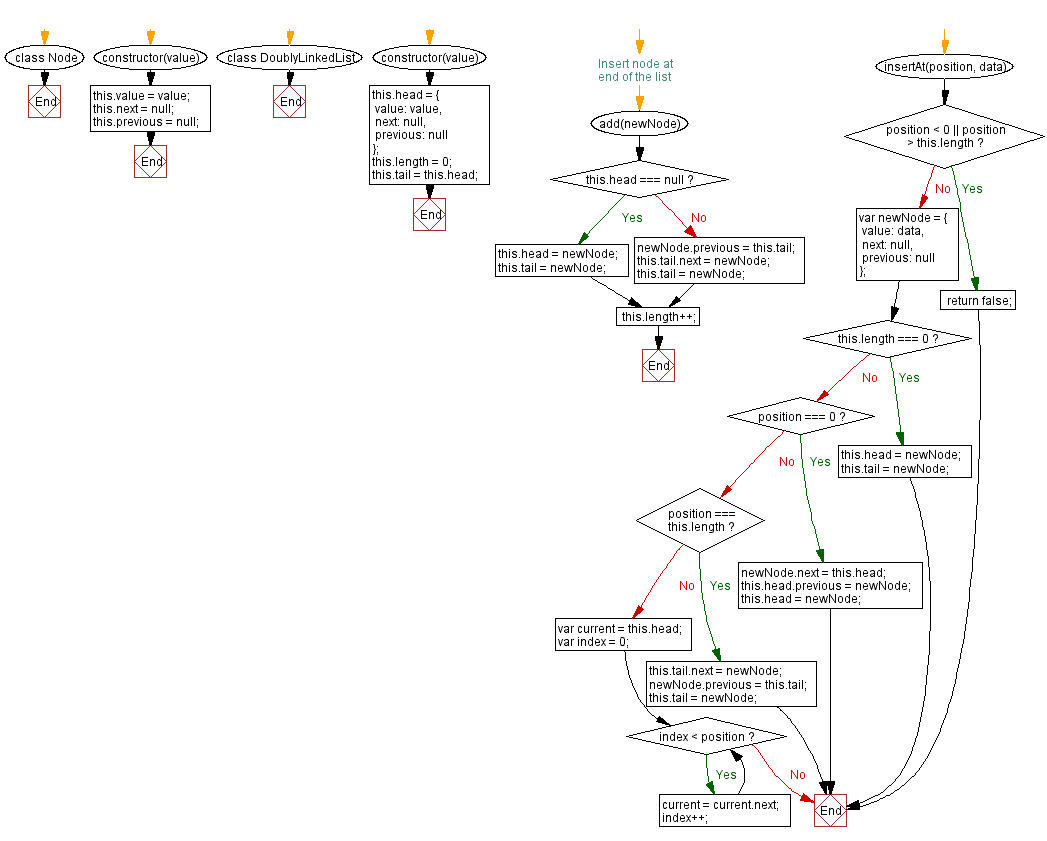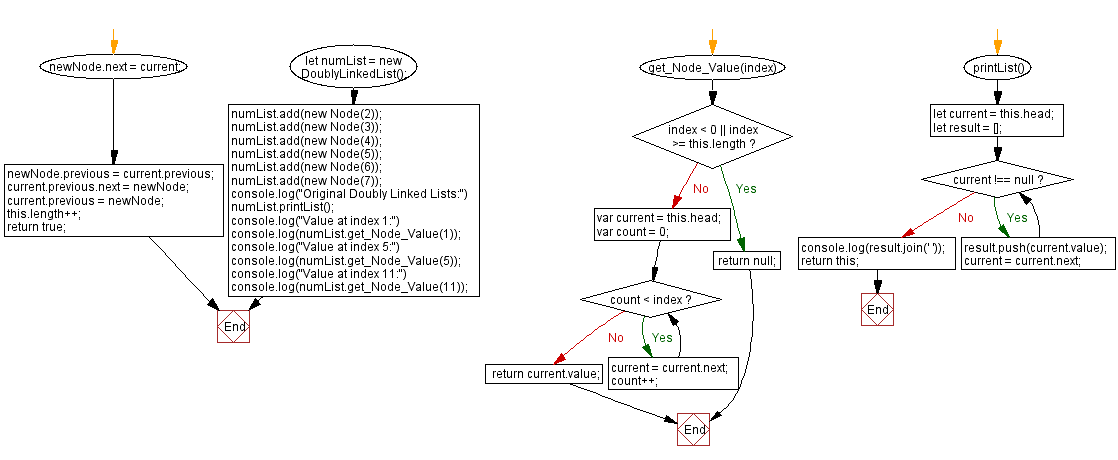# JavaScript Exercises: Get the value of a node at a given position in a Doubly Linked List

## JavaScript Data Structures: Exercise-8 with Solution

Write a JavaScript program to get the value of a node at a given position in a Doubly Linked List.

Sample Solution:

JavaScript Code:

`````` class Node {
constructor(value) {
this.value = value;
this.next = null;
this.previous = null;
}
}
constructor(value) {
value: value,
next: null,
previous: null
};
this.length = 0;
}
// Insert node at end of the list
this.tail = newNode;
}
else
{
newNode.previous = this.tail;
this.tail.next = newNode;
this.tail = newNode;
}
this.length++;
}
insertAt(position, data) {
if (position < 0 || position > this.length) {
return false;
}
var newNode = {
value: data,
next: null,
previous: null
};
if (this.length === 0) {
this.tail = newNode;
} else if (position === 0) {
} else if (position === this.length) {
this.tail.next = newNode;
newNode.previous = this.tail;
this.tail = newNode;
} else {
var index = 0;
while (index < position) {
current = current.next;
index++;
}
newNode.next = current;
newNode.previous = current.previous;
current.previous.next = newNode;
current.previous = newNode;
}
this.length++;
return true;
}
get_Node_Value(index) {
if (index < 0 || index >= this.length) {
return null;
}
var count = 0;
while (count < index) {
current = current.next;
count++;
}
return current.value;
}
printList() {
let result = [];
while (current !== null) {
result.push(current.value);
current = current.next;
}
console.log(result.join(' '));
return this;
}
}
numList.printList();
console.log("Value at index 1:")
console.log(numList.get_Node_Value(1));
console.log("Value at index 5:")
console.log(numList.get_Node_Value(5));
console.log("Value at index 11:")
console.log(numList.get_Node_Value(11));
```
```

Sample Output:

```"Original Doubly Linked Lists:"
" 2 3 4 5 6 7"
"Value at index 1:"
2
"Value at index 5:"
6
"Value at index 11:"
null
```

Flowchart:Live Demo:

See the Pen javascript-doubly-linked-list-exercise-8 by w3resource (@w3resource) on CodePen.

Improve this sample solution and post your code through Disqus

Linked List Previous: Insert a new node at the end of a Doubly Linked List.

What is the difficulty level of this exercise?

Test your Programming skills with w3resource's quiz.

﻿

## JavaScript: Tips of the Day

Shorten an array using its length property

A great way of shortening an array is by redefining its length property.

```let array = [0, 1, 2, 3, 4, 5, 6, 6, 8, 9]
array.length = 4
// Result: [0, 1, 2, 3]
```

Important to know though is that this is a destructive way of changing the array. This means you lose all the other values that used to be in the array.

Ref: https://bit.ly/2LBj213